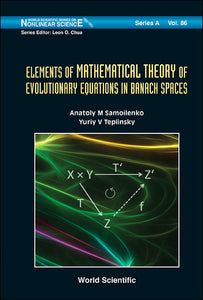# Elements Of Mathematical Theory Of Evolutionary Equations In Banach Spaces

Anatoliy M Samoilenko

Format: Print Book

ISBN: 9789814434829

• SGD 201.16
Unit price per
Tax included.

Evolutionary equations are studied in abstract Banach spaces and in spaces of bounded number sequences. For linear and nonlinear difference equations, which are defined on finite-dimensional and infinite-dimensional tori, the problem of reducibility is solved, in particular, in neighborhoods of their invariant sets, and the basics for a theory of invariant tori and bounded semi-invariant manifolds are established. Also considered are the questions on existence and approximate construction of periodic solutions for difference equations in infinite-dimensional spaces and the problem of extendibility of the solutions in degenerate cases. For nonlinear differential equations in spaces of bounded number sequences, new results are obtained in the theory of countable-point boundary-value problems.The book contains new mathematical results that will be useful towards advances in nonlinear mechanics and theoretical physics.

Format: Hardcover
No of Pages: 408
Imprint: World Scientific
Publication date: 20130506
Series: World Scientific Series On Nonlinear Science Series A